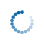# Math Is Fun Forum

Discussion about math, puzzles, games and fun.   Useful symbols: ÷ × ½ √ ∞ ≠ ≤ ≥ ≈ ⇒ ± ∈ Δ θ ∴ ∑ ∫  π  -¹ ² ³ °

You are not logged in.

## #1 2015-09-27 05:52:39

denis_gylaev
MemberRegistered: 2015-03-19
Posts: 66

### More Circles

Point Y is on a circle and point P lies outside the circle such that \overline{PY} is tangent to the circle. Point A is on the circle such that segment \overline{PA} meets the circle again at point B. If PA = 15 and PY = 9, then what is AB?

Chords \overline{WY} and \overline{XZ} of a circle are perpendicular. If XV = 4, WV = 3, and VZ = 9, then find YZ.

Chords \overline{AB} and \overline{XY} of a circle meet at T. If XT = 4, TY = 6, and AT = 2TB, then what is AB?

Offline

## #2 2015-09-27 19:41:25

BobRegistered: 2010-06-20
Posts: 9,425

### Re: More Circles

hi denis_gylaev

There's a theorem in geometry that covers all three questions here.

I'll start with the middle question.  V is the point where WY and XZ cross.

In triangles XWV and YZV, angle XWY = XZY (using angle props. of a circle) and WVX = ZVY (= 90**) so the triangles are similar.  Thus:

In the third question the crossing point is outside the circle, but the theorem still holds.

In triangles TXA and TBY, angle T is common to both, angle XAB = XYB. So the triangles are similar and

Question 1.  If you rename point T as point P, then PB.PA = PY.PX

Let X approach Y.  Then the equation becomes, in the limit,  PB.PA = PY^2

Bob

**By vertically opposite angles this would still be true even if the angle wasn't 90.  But you need 90 in order to use Pythagoras theorem to complete the problem.

Children are not defined by school ...........The Fonz
You cannot teach a man anything;  you can only help him find it within himself..........Galileo Galilei
Sometimes I deliberately make mistakes, just to test you!  …………….BobOffline